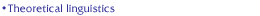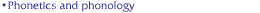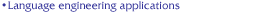## Outline

Many of the language and speech processing technologies use statistical formulations based on important basic concepts of statistical pattern classification. As a matter of fact, current state-of-the-art speech recognition systems are based on stochastic models, which parameters are automatically trained on large corpora of acoustic and text databases. More specifically, speech units (words, syllable or phones) are usually represented in terms of Hidden Markov Models (HMM), a particular case of stochastic finite state automaton, while the syntactical constraints are usually approximated by stochastic grammars (N-grams). Consequently, this topic area covers the basic concepts and theories underlying statistical pattern classification and that will be necessary to students to understand the techniques underlying the prevailing approaches to language and speech processing.

Material on the application of statistical pattern classification to Automatic Speech Recognition is also covered under the Language Engineering Applications area.

## Topics

• Statistical pattern recognition
• Bayes'rule, minimum error classification
• Maximum a posteriori and maximum likelihood classification
• Likelihood Estimation-Maximization (EM) algorithm
• Statistically based discriminant (linear and nonlinear discriminant functions)
• Artificial neural networks
• Vector quantization (K-means, KNN, etc)
• Decision trees, regression trees
• Feature extraction and analysis (linear discriminant analysis, principal component analysis)
• Stochastic finite state automata and discrete Markov models
• Definition and operation of stochastic finite state automata
• Discrete Markov models (parametrization and probability estimation)
• Examples of applications
• Hidden Markov models (HMM)
• Definition, parametrization and hypotheses
• Estimation of model probabilities (Viterbi and forward recurrences), dynamic programming
• Estimation of HMM parameters
• HMMs for classification of (piecewise stationary) temporal sequences

## References

1. Bishop, Christopher M. (1995) Neural Networks for Pattern Recognition. Oxford University Press.
2. Papoulis, A. (1965) Probability, Random Variables, and Stochastic Processes, McGraw-Hill.
3. Duda, R., and Hart, P. (1973) Pattern Classification and Scene Analysis, Wiley-Interscience.
4. Therrien, C. (1989) Decision, Estimation and Classification, John Wilwey.
5. Jelinek, F. (1998) Statistical Method for Speech Recognition, MIT Press, Cambridge Massachusetts.
|Overview| |Course Content| |Members| |Participate| |Search| |Questions| |School|
|Theoretical Linguistics| |Natural Language Processing| |Phonetics and Phonology| |Cognitive Models for Speech Language Processing| |Speech Signal Processing| |Pattern Recognition| |Language Engineering Applications|